## The Perturbation Series

Assume that the energy eigenvalue problem for the Hamiltoniancan be solved exactly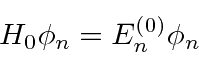but that the true Hamiltonian has a small additional term or perturbation.The Schrödinger equation for the full problem is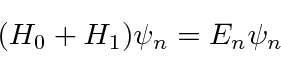Presumably this full problem, like most problems, cannot be solved exactly. To solve it using a perturbation series,we will expand both our energy eigenvalues and eigenstates in powers of the small perturbation.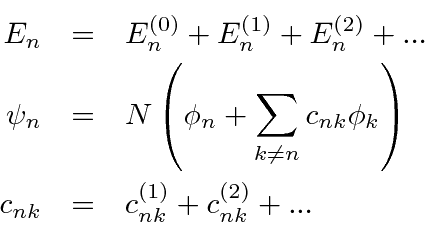where the superscript (0), (1), (2) are the zeroth, first, and second order terms in the series.is there to keep the wave function normalized but will not play an important role in our results.

By solving the Schrödinger equation at each order of the perturbation series, we compute the corrections to the energies and eigenfunctions.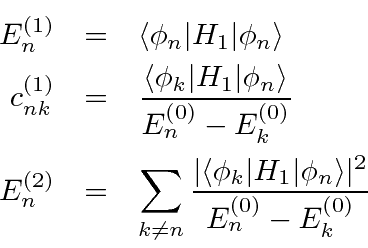So the first order correction to the energy of theeigenstate,, is just the expectation value of the perturbation in the unperturbed state. The first order admixture ofin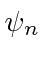,, depends on a matrix element and the energy difference between states. The second order correction to the energy,, has a similar dependence. Note that the higher order corrections may not be small if states are nearby in energy.

The application of the first order perturbation equations is quite simple in principal. The actual calculation of the matrix elements depends greatly on the problem being solved.

* Example: H.O. with anharmonic perturbation ().*

Sometimes the first order correction to the energy is zero. Then we will need to use the second order termto estimate the correction. This is true when we apply an electric field to a hydrogen atom.

* Example: Hydrogen Atom in a E-field, the Stark Effect.*

We will exercise the use of perturbation theory in section 23 when we compute the fine structure, and other effects in Hydrogen.

Jim Branson 2013-04-22